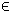## 6MIQQ Problem

The general formulation in TOMLAB for a mixed-integer quadratic programming problem with quadratic constraints is:

 min x
f(x) =
1
2
xT F x + cT x
s/t xL x xU
bL Ax bU
xT Q(i) x + a(i)T x rU(i), i=1,…,nqc
xi integer   iI
(7)
where c, x, xL, xU, a(i)Rn, F, Q(i)Rn× n, ARm× n and bL,bURm. rU(i) is a scalar. The variables xI, the index subset of 1,...,n, are restricted to be integers.

The following file illustrates how to solve a MIQQ problem in TOMLAB.

File: tomlab/quickguide/miqqQG.m

Open the file for viewing, and execute miqqQG in Matlab.
```% miqqQG is a small example problem for defining and solving
% using the TOMLAB format.

Name = 'MIQQ Test Problem 1';
f_Low = -1E5;
x_opt = [];
f_opt = [];
IntVars = [0 0 1];

F   = [2 0 0;0 2 0;0 0 2];
A   = [1 2 -1;1 -1 1];
b_L = [4 -2]';
b_U = b_L;
c   = zeros(3,1);

x_0 = [0 0 0]';
x_L = [-10 -10 -10]';
x_U = [10 10 10]';
x_min = [0 0 -1]';
x_max = [2 2 1]';

qc(1).Q = speye(3,3);
qc(1).a = zeros(3,1);
qc(1).r_U = 3;

qc(2).Q = speye(3,3);
qc(2).a = zeros(3,1);
qc(2).r_U = 5;

Prob = miqqAssign(F, c, A, b_L, b_U, x_L, x_U, x_0, qc,...
IntVars, [], [], [],...
Name, [], [],...
x_min, x_max, f_opt, x_opt);

Result = tomRun('cplex', Prob, 1);
% Result = tomRun('minlpBB', Prob, 1);```